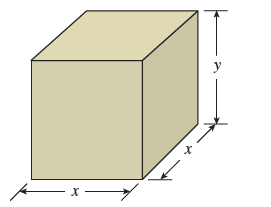Bri C.

# determine the dimensions (in ft) of the box that can be constructed at minimum cost. (Refer to the figure below.)

A rectangular box is to have a square base and a volume of 20 ft3. If the material for the base costs $0.19/ft2, the material for the sides costs$0.06/ft2, and the material for the top costs \$0.11/ft2, determine the dimensions (in ft) of the box that can be constructed at minimum cost. (Refer to the figure below.)x= ____ft

y= ____ft